# Integral equations, numerical methods

Methods for finding approximate solutions of integral equations.

It is required to find the solution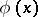of a one-dimensional Fredholm equation of the second kind,(1)

whereis continuous on,is a numerical parameter and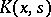is continuous on.

Suppose thatis not an eigen value of. Then equation (1) has a unique solution, which is continuous on. Under these conditions one can give the following methods for obtaining an approximate solution.

## First method.

Letandbe finite numbers. The integral in (1) is replaced by an integral sum over a grid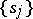,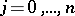, while the variabletakes the values. One then obtains a system of linear algebraic equations with respect to the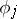,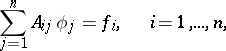(2)

where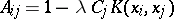, and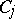are the coefficients of the quadrature formula by means of which the integral in (1) is replaced by the integral sum. For sufficiently large, the system (2) has a unique solution. As an approximate solution to (1) one can take the function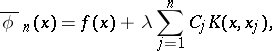since asand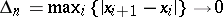, the sequence of functions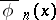converges uniformly onto the required solution of equation (1). See .

When replacing the integral by a quadrature formula, one has to bear in mind that the greater the precision of the quadrature formula used, the greater the smoothness of the kernel and solution (and hence also) needs to be.

In the case when the range of integrationis infinite, it is replaced by a finite interval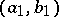by using a priori information concerning the behaviour of the required solution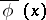for large values of. The equation so obtained is then approximately solved by the above method. Alternatively, by a change of the integration variable the range of integration is reduced to a finite range. As another alternative, quadrature formulas for an infinite range can be applied.

## Second method.

In equation (1) the kernelis replaced by a degenerate kernel approximating it: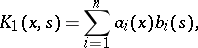in which the functionsare linearly independent. The equation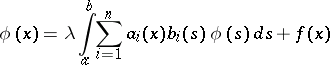(3)

obtained in this way has a solution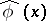of the form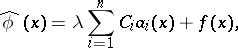(4)

in which the constantshave to be determined. On substituting the functioninto equation (3) and comparing the coefficients at the functions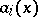one obtains a system of linear algebraic equations for the:where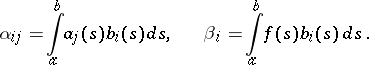Having determined the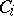from the above system and by substituting them in (4) one obtains the function, which is taken as the approximate solution of (1), since for a sufficiently good approximation of the kernelby a degenerate kernel the solution of equation (3) differs by an arbitrarily small amount from the required solutionon any interval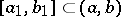, as well as onin the case when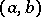is a finite interval (see , ).

## Third method.

As approximate solutions one takes functionsobtained by iteration based on the formula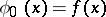; the sequenceconverges uniformly to the required solution as, provided that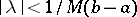, where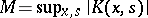. Convergence ofto the exact solution also holds for kernels with an integrable singularity (see ). Estimates of the errors of these methods are given in . In  the question is considered of the minimum number of arithmetical operations required in order to obtain an approximate value of the integral with a prescribed precision. The solution of this problem is equivalent to finding the size of the minimal error of an approximate solution of the problem under a prescribed number of arithmetical operations.

For the solution of Fredholm integral equations of the first kind special methods need to be applied, since these problems are ill-posed. If in equation (1)is one of the eigen values of the kernel, then the problem of finding a solution of (1) is ill-posed and requires special methods (see Ill-posed problems).

Non-linear equations of the second kind are usually solved approximately by an iterative method (see ).

The Galerkin method for obtaining approximate solutions of linear and non-linear equations is also used.

Similar methods can also be applied for obtaining approximate solutions of multi-dimensional Fredholm integral equations of the second kind. However, their numerical implementation is more complicated. See  for cubature formulas for the approximate computation of multiple integrals and their error estimates. A Monte-Carlo method of approximate numerical computation of multiple integrals is discussed in .